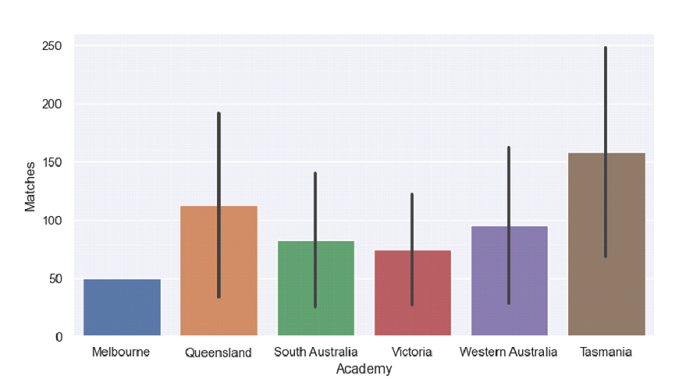# Python Pandas - Draw a bar plot and show standard deviation of observations with Seaborn

Bar Plot in Seaborn is used to show point estimates and confidence intervals as rectangular bars. The seaborn.barplot() is used for this. Display Standard Deviation of Observations using confidence interval ci parameter value sd.

Let’s say the following is our dataset in the form of a CSV file − Cricketers2.csv

At first, import the required libraries −

import seaborn as sb
import pandas as pd
import matplotlib.pyplot as plt

Load data from a CSV file into a Pandas DataFrame −

dataFrame = pd.read_csv("C:\Users\amit_\Desktop\Cricketers2.csv")

Plotting bar plot with Academy and Matches. Display Standard Deviation of Observations using confidence interval parameter value "sd" −

sb.barplot(x = "Academy", y = "Matches",data = dataFrame, ci = "sd")

## Example

Following is the code −

import seaborn as sb
import pandas as pd
import matplotlib.pyplot as plt

# Load data from a CSV file into a Pandas DataFrame

sb.set_theme(style="darkgrid")

# plotting bar plot with Academy and Matches
# Display Standard Deviation of Observations using confidence interval parameter value "sd"
sb.barplot(x = "Academy", y = "Matches",data = dataFrame, ci = "sd")

# display
plt.show()

## Output

This will produce the following output −